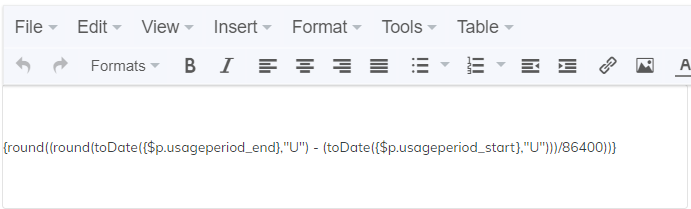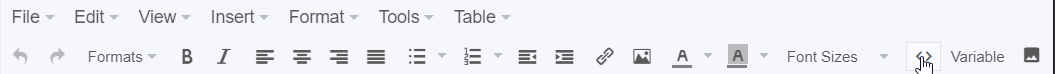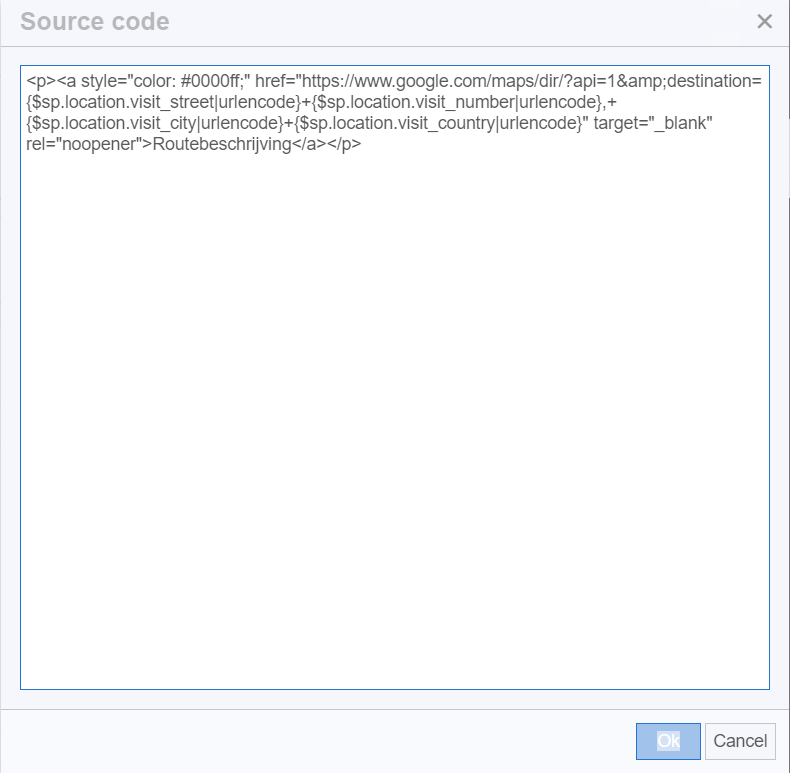# Document template custom code snippets

To further personalize your documents outside the built-in options of our editor, you can use custom PHP, HTML, and CSS. Creating these custom solutions for you can be done as part of our Professional services. However, we’ve collected several commonly requested/used code snippets for you below.

Some of these snippets require you to enter some data, for example, a price manually. We will indicate this by styling the required data as a variable as such:

This is some code to add a certain amount of money to your reminders, the amount of money will be shown as \$AMOUNT. So the code snippet will be:

Reminder + \$AMOUNT€

`{sumprice({\$f.invoice.price_invat},\$AMOUNT)}`

If you would like to fill this in with 5€ you would replace \$AMOUNT with 5 like this:

`{sumprice({\$f.invoice.price_invat},5)}`

# PHP

These code snippets can be copy/pasted into a text module in Rentman like this:## List

Calculate the amount of days in your Usage Period:

`{round((round(toDate({\$p.usageperiod_end},"U") - (toDate({\$p.usageperiod_start},"U")))/86400))}`

Calculate the amount of days in your Usage Period and show 0 days a 1 day

```{iff {round(({\$p.usageperiod_end,U}-{\$p.usageperiod_start,U})/86400)} == 0}1
day {/iff} {iff {round(({\$p.usageperiod_end,U}-{\$p.usageperiod_start,U})/86400)}
!= 0 }{round(({\$p.usageperiod_end,U}-{\$p.usageperiod_start,U})/86400)} day(s)
{/iff}```

Round digits to 2 decimal places

`{\$VARIABLE|round:2}`

\$VARIABLE: The numeric variable that you would like rounded.

% on top of price

`{sumprice({\$PRICE},{multiplyprice({\$PRICE},-\$PERCENTAGE)})}`

\$PRICE: The price variable that you would like to increase.

\$PERCENTAGE: The percentage that you would like to increase it by.

Note:The ‘-’ at the end is required to make this work.

Substract price 1 from price 2

```{sumprice({\$PRICE1},{multiplyprice({\$PRICE2},-1)})}
```

\$PRICE1: The price variable that you would like to subtract.

\$PRICE2: The price variable that you would like \$PRICE1 to be subtracted from.

Rounding price on 5 cents

`{math equation="{round({math equation="({removePrice({\$PRICE})}/0.05)"})}* 0.05"}`

\$PRICE: The price variable that you would like to round.

Belgian “gestructureerde mededeling”

8 digit invoicenumber + extra number:

`{\$f.number|substr:0:8}/28{assign var="code" value=" {\$f.number|substr:0:8}28"}/{\$code % 97}`

Customer code + project number:

```{assign var = "code1" value =\$f.number|str_pad:3:"0":STR_PAD_LEFT|substr:0:3}
{assign var = "code" value ="\$code1\$code2\$code3"}
{assign var = "check" value =\$code % 97}
+++{\$code1}/{\$code2}/{\$code3}{\$check|replace:'0':'97'}+++ ```

Date formats

`{\$DATE,Y}-{\$DATE|date_format:"%m"}-{\$DATE|date_format:"%d"}`

\$DATE: The date variable that you want to display This is an example of an American date format; the added variable modifiers here (%d and %m) can be replaced to show a wide variety of information. Please see the full list here.

# HTML

To add HTML to your documents you can edit a text module and open the source code with the <> icon.After this you can paste one of the following code snippets:

Get route to location of main project as link

```<a style="color: #0000ff;" href="https://www.google.com/maps/dir/?api=1&destination={\$sp.location.visit_street|urlencode}+{\$sp.location.visit_number|urlencode},+{\$sp.location.visit_city|urlencode}+{\$sp.location.visit_country|urlencode}" target="_blank" rel="noopener">Routebeschrijving</a>
```

QR to open subproject in the app

```  <div><img src="https://qr-generator.io/?cht=qr&chs=150x150&chl=project_{\$p.id}:subproject_{\$sp.id}&chld=H|0" width="90" height="90" /></div>
```

Make a QR for anything you’d like

`  <div><img src="https://qr-generator.io/?cht=qr&chs=150x150&chl=\$VARIABLE&chld=H|0" width="90" height="90" /></div>`

\$VARIABLE = Whatever information the QR needs to convey.